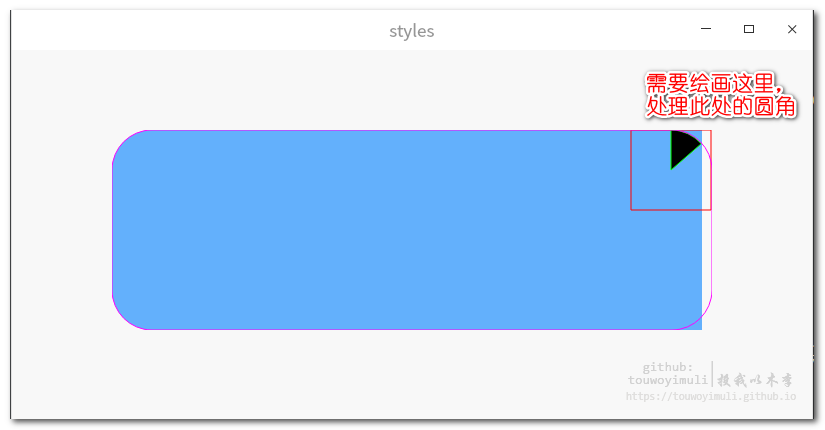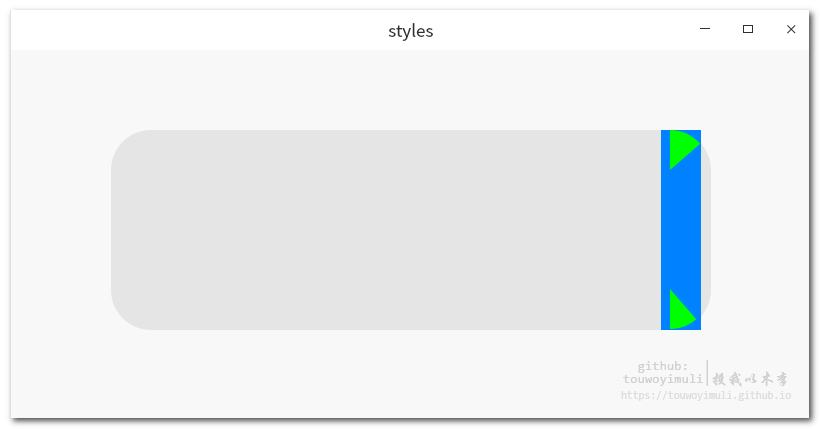[TOC]

## 解决方法:其中需要计算角度

## 绘画扇形drawPie()：

void QPainter::drawPie(const QRect &, int a, int alen)

``p->drawPie(topPointRect,41 * 16, (90 - 41) * 16);``

## 反三角计算角度qAcos()：

``#include <QtMath>``

qreal qAcos(qreal v)
Returns the arccosine of v as an angle in radians. Arccosine is the inverse operation of cosine.

``qreal raw = qAcos(30 * 1.0 / 40);   //=41.4096弧度``

## 弧度和角度转换公式:

π所对应的宏为:

``//M_PI	The ratio of a circle's circumference to diameter, π``

``````qreal raw = qAcos(30 * 1.0 / 40);
int startRadius = raw * 180 / M_PI;